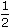# Electronics and Communication Engineering - Electronic Devices and Circuits

36.

For a junction FET in the pinch off region as the drain voltage is increased, the drain current

 A. becomes zero B. abruptly decreases C. abruptly increases D. remains constant

Answer: Option D

Explanation:

No answer description available for this question. Let us discuss.

37.

To avoid thermal runaway in the design of an analog circuit, the operating point of the BJT should be such that it satisfies the condition.

 A. VCE =VCC B. VCE ≤VCC C. VCE ≥VCC D. VCE ≤ 0.78 VCC

Answer: Option B

Explanation:

No answer description available for this question. Let us discuss.

38.

For a MOS capacitor fabricated on a P-type semiconductor, strong inversion occurs when

 A. surface potential is equal to Fermi potential B. surface Potential is greater than Fermi potential C. surface potential is - ve and equal to Fermi potential in magnitude D. surface potential is + ve and equal to twice the Fermi potential

Answer: Option D

Explanation:

No answer description available for this question. Let us discuss.

39.

The primary function of a clamper circuit is to

 A. suppress variations in signal voltage B. raise +ve half cycle to the signal C. Lower -ve half cycle of the signal D. introduce a dc level into an a.c. signal

Answer: Option B

Explanation:

No answer description available for this question. Let us discuss.

40.

Breakdown in dielectric may be

 A. electrical breakdown B. thermal breakdown C. electrochemical breakdown D. any of the above

Answer: Option D

Explanation:

No answer description available for this question. Let us discuss.

#### Current Affairs 2021

Interview Questions and Answers